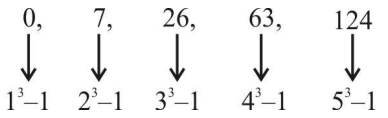Home » Verbal Reasoning » Number Series » Question

#### Number Series

Direction: What will come in the place of question mark (?) in the following number.

1. 0, 7, 26, 63, ?
1. 125
2. 126
3. 217
4. 124
5. None of these
##### Correct Option: D

The given series is in the below pattern,we can see that
Next term ( Tn ) = n3 - 1 { where , putting n = 1 , 2 , 3 , 4 , 5 respectively }
First term = T1 = 13 - 1 = 0
Second term = T2 = 23 - 1 = 7
Similarly we can find the all others terms
Third term = 26
Fourth term = 63
Fifth term( ? )= 124
Hence we get the value of ? = 124